# Parallel lines and transversals

0/1
##### Introduction
###### Lessons
1. Relationships between lines and angles
0/8
##### Examples
###### Lessons
1. Find the missing values and then explain your reasoning.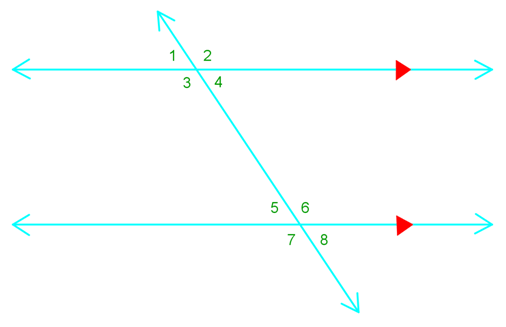1. $\angle 6$ $\cong$ ?
2. $\angle 2$ $\cong$ ?
3. if m $\angle 8$ = 60°, m $\angle 1$ = ?
4. $\angle 3$ and $\angle ?$ Are supplementary
2. Find the value of x.

1.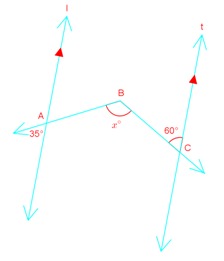2.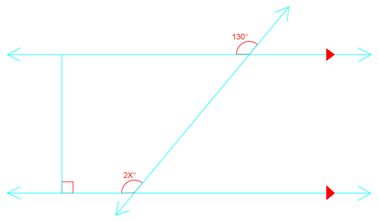3.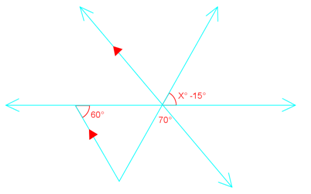3. Write a two-column proof.
Given: $\overline {AB}$ $\parallel$ $\overline {CD}$
Prove: $\angle 1$ $\cong$ $\angle 2$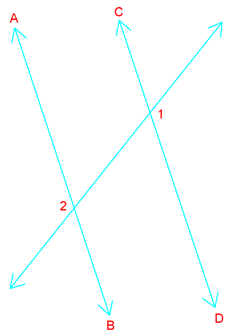###### Free to Join!
StudyPug is a learning help platform covering math and science from grade 4 all the way to second year university. Our video tutorials, unlimited practice problems, and step-by-step explanations provide you or your child with all the help you need to master concepts. On top of that, it's fun - with achievements, customizable avatars, and awards to keep you motivated.
• #### Easily See Your ProgressWe track the progress you've made on a topic so you know what you've done. From the course view you can easily see what topics have what and the progress you've made on them. Fill the rings to completely master that section or mouse over the icon to see more details.
• #### Make Use of Our Learning Aids###### Practice Accuracy

See how well your practice sessions are going over time.

Stay on track with our daily recommendations.

• #### Earn Achievements as You LearnMake the most of your time as you use StudyPug to help you achieve your goals. Earn fun little badges the more you watch, practice, and use our service.
• #### Create and Customize Your AvatarPlay with our fun little avatar builder to create and customize your own avatar on StudyPug. Choose your face, eye colour, hair colour and style, and background. Unlock more options the more you use StudyPug.

## Parallel lines

What are parallel lines? They are lines that are equidistant (the same distance apart) from each other but never meet. They also point in the same direction.

When a set of parallel lines gets cut by another line, that other line is called a transversal. Having this transversal line paves the way for a variety of helpful information that we can derive from the parallel lines' angles.

## What are corresponding angles

When we've got a line with a transversal through it, we can identify corresponding angles from the two parallel lines that are the same. Therefore, corresponding angles are equal. This is the corresponding angles definition. We'll have a chart below to summarize this and the following concept at the end with visual depictions of what these terms mean.

## What are alternate interior angles

Alternate interior angles are found on the opposite sides of the transversal but inside the two lines. These angles are on the interior of the lines that cross, but on alternate sides of the transversal. This is the alternate interior angles definition.

Here's a summary chart of the above concepts, plus a few more pairs of angles that come about due to parallel lines and a transversal. Unlike regular pairs of lines and angles, these ones have special rules to their angles based off the parallel lines. They'll be very useful in helping you solve questions related to pairs of angles.

## Example questions

Question 1:

Find the missing values and then explain your reasoning.

a) angle 6 $\cong$ ?

b) angle 2 $\cong$ ?

c) if m angle 8 = 60°, m angle 1 = ?

d) angle 3 and angle ? are supplementary

Solutions:

a) angle 6 $\cong$ ?

Angle 6 is congruent to angle 2 due to the corresponding angle rule.

Angle 6 is also congruent to angle 7 due to vertical angle congruence.

Lastly, angle 6 is congruent to angle 3 because of the corresponding angle rule between angle 3 and angle 7.

b) angle 2 $\cong$ ?

Angle 2 is equalled to angle 6 since they're corresponding angles.

Angle 2 is also congruent to angle 3 due to vertical angle congruence.

Lastly, angle 2 is congruent to angle 7 based on the corresponding angle rule or alternate exterior angle theorem.

c) if m angle 8 = 60°, m angle 1 = ?

We get that m angle 1 = 60°. This is based on the alternate exterior angle theorem.

d) angle 3 and angle ? are supplementary

Supplementary means that the sum the two angles = 180°

So angle 3 + angle 5 is supplementary due to the consecutive interior angle theorem.

In addition, angle 8, angle 1, angle 4 are also supplementary to angle 3.

Question 2:

Find the value of x.

Solution:

Angle 130° = 2x°

So,

130 = 2x

65° = x

Question 3:

Write a two-column proof.

Given: AB||CD

Prove: angle 1 $\cong$ angle 2

Solution:

 Statements reasons AB||CD given angle 3 $\cong$ angle 1 Vertical angle congruence theorem angle 3 $\cong$ angle 2 Corresponding angle postulate angle 1 $\cong$ angle 2 Transitive property of congruence

Take a look at this interactive online diagram to help you see the angle relationships that exist when there is a set of parallel lines and a transversal.

To move further into this concept, learn more about parallel line equation and how to deal with a combination of parallel and perpendicular line equations.